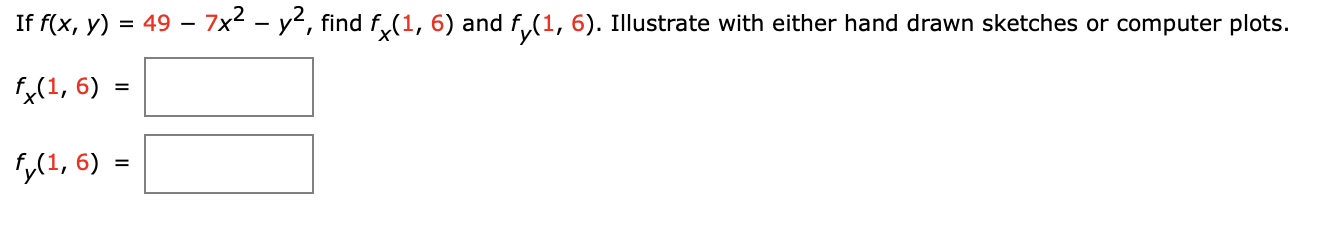# Question If f(x, y) = 49 – 7x2 - y2, find f{(1, 6) and fy(1, 6). Illustrate with either hand drawn sketches or computer plots. (1, 6) = fy(1,6) =